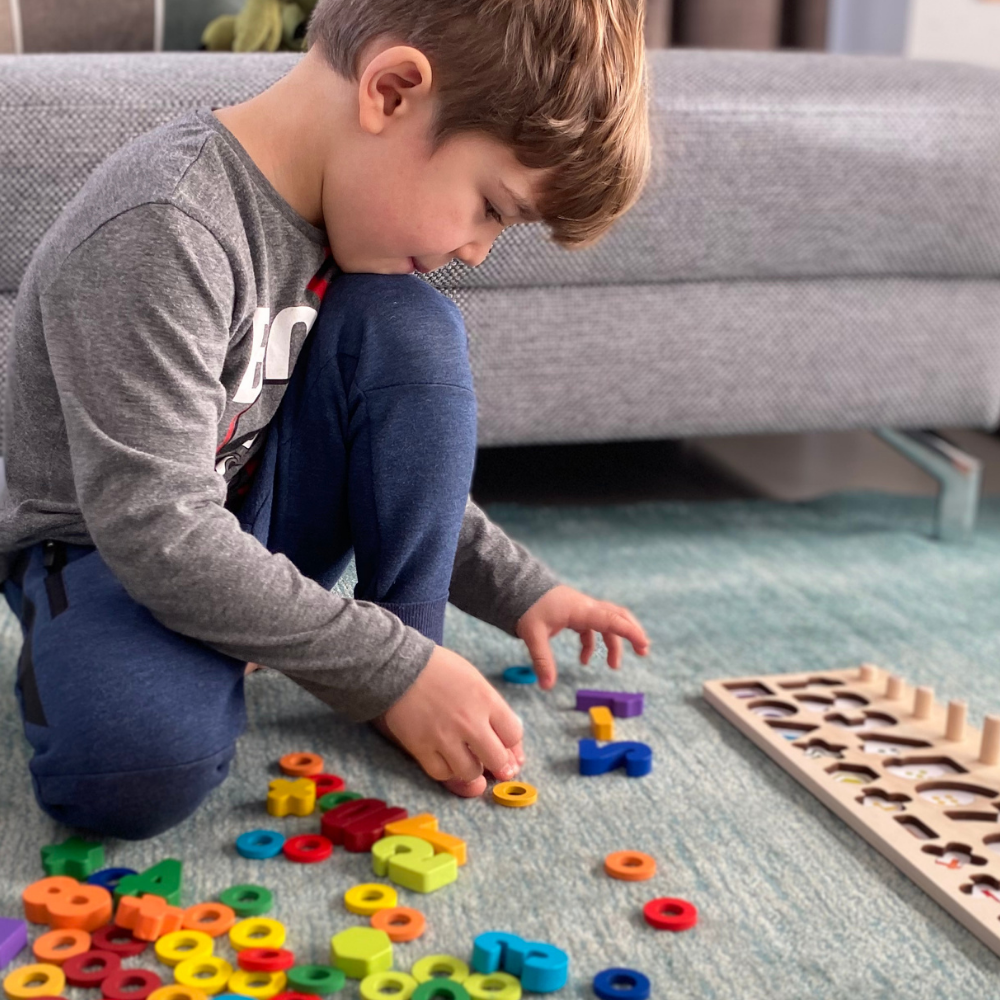# Game idea: Color mathAge: From 6 years

Players: From 1 child

What do you need?
The Number puzzle

How is it played?
Create possible equations with the equal, plus, minus, multiplication and divided signs and practice your first mathematical operations! To do this, place the rings in the correct amount above or below the numbers to check whether the calculation is correct. What types of calculations do you already know? Do you know what 5+3 is? Or 8-2? Do you already know 2x5? And maybe also 9:3? Have fun laying and overlaying!

What is promoted?
Basic mathematical understanding, focus, concentration, logical thinking